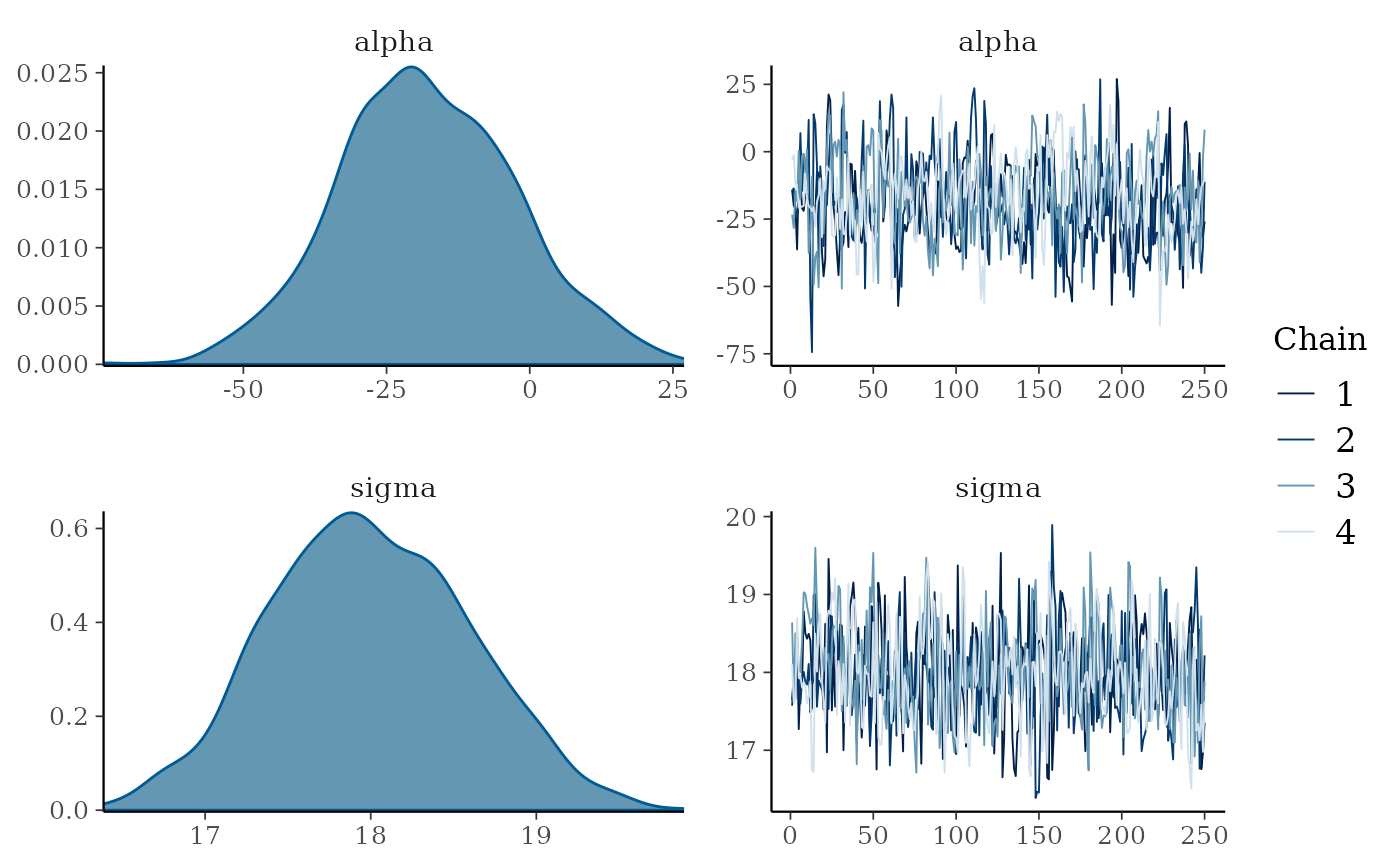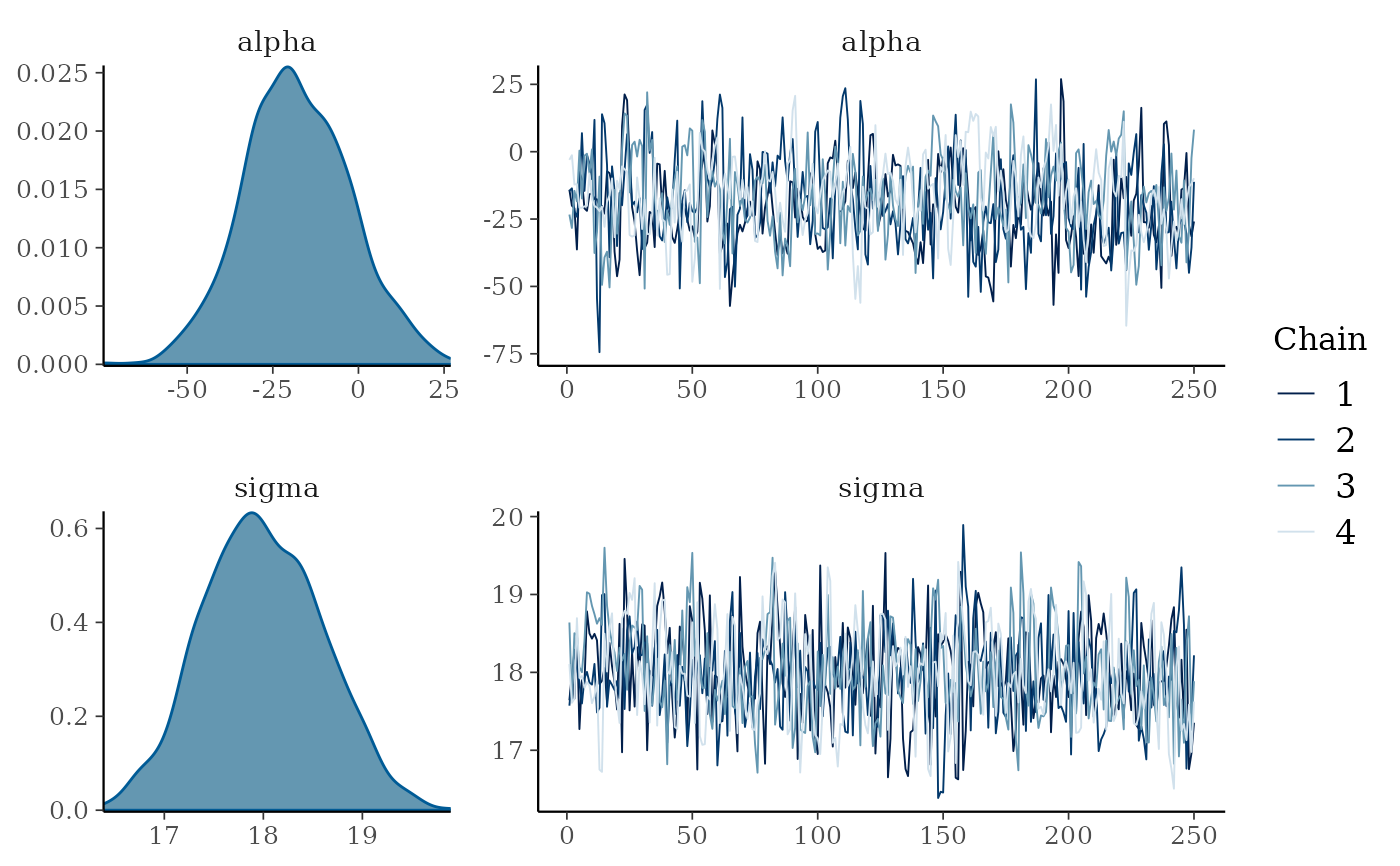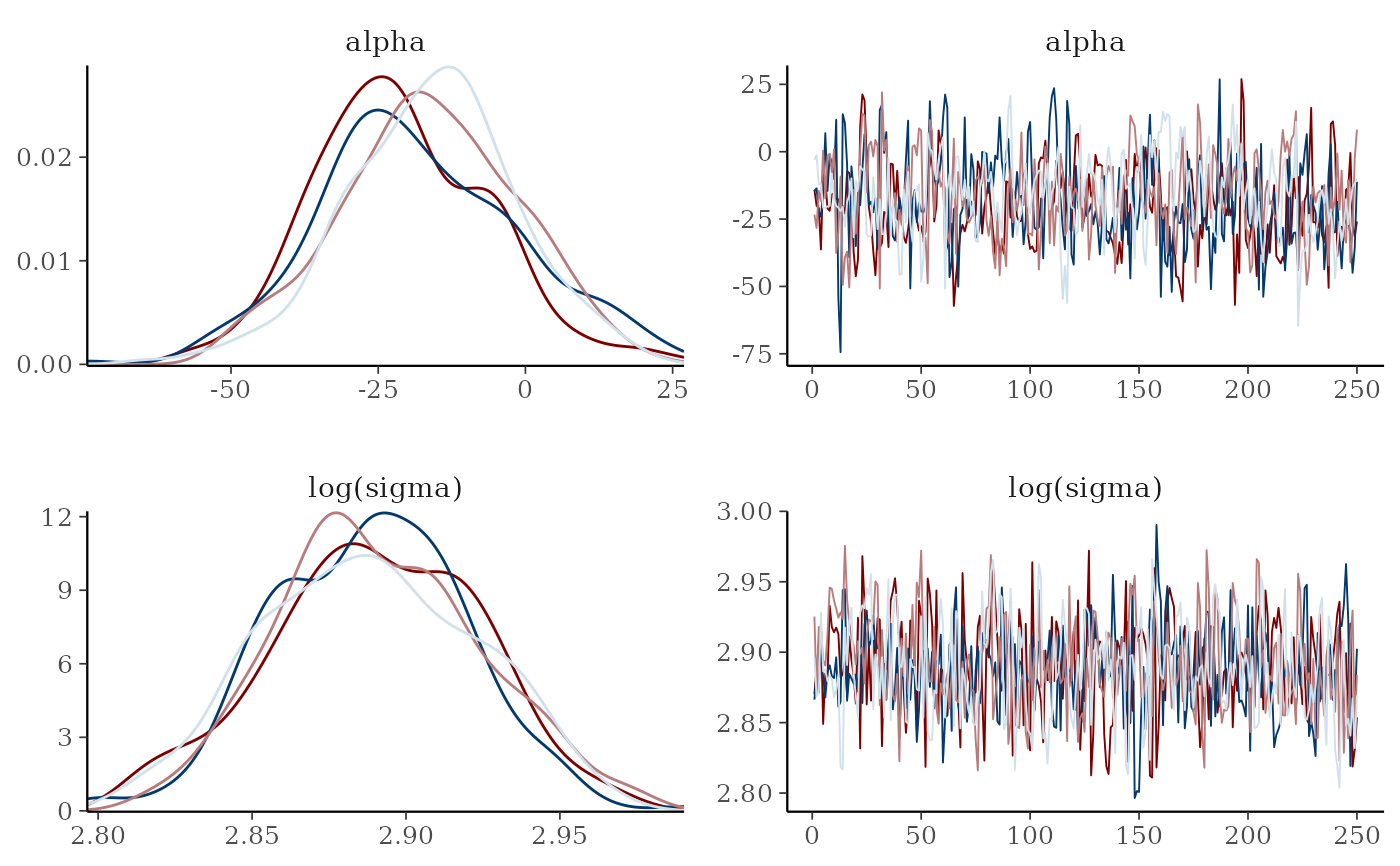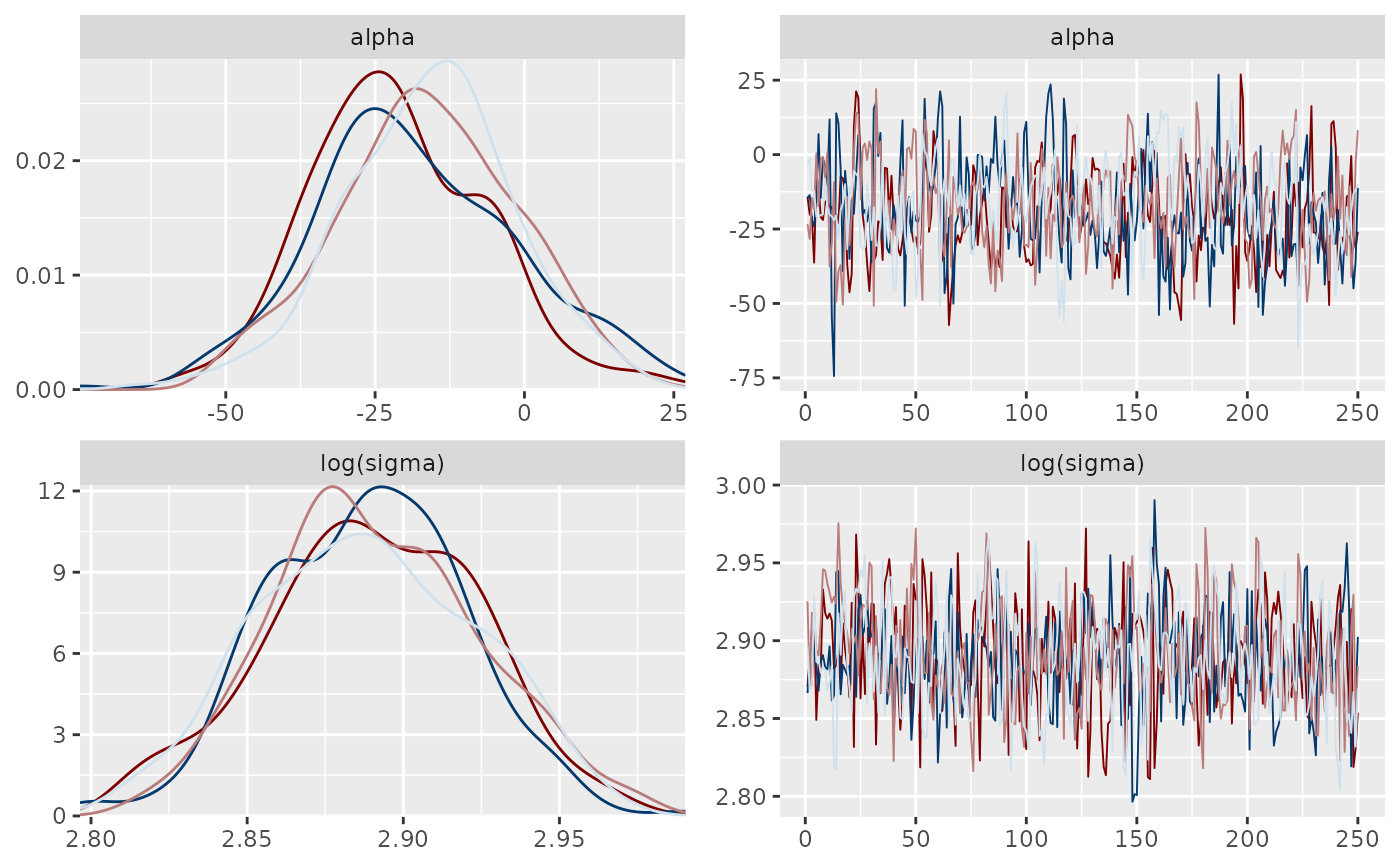Combination plots

mcmc_combo(x, combo = c("dens", "trace"), ..., widths = NULL, gg_theme = NULL)

## Arguments

x

An object containing MCMC draws:

• A 3-D array, matrix, list of matrices, or data frame. The MCMC-overview page provides details on how to specify each these.

• A draws object from the posterior package (e.g., draws_array, draws_rvars, etc.).

• An object with an as.array() method that returns the same kind of 3-D array described on the MCMC-overview page.

combo

A character vector with at least two elements. Each element of combo corresponds to a column in the resulting graphic and should be the name of one of the available MCMC functions (omitting the mcmc_ prefix).

...

Arguments passed to the plotting functions named in combo.

widths

A numeric vector the same length as combo specifying relative column widths. For example, if the plot has two columns, then widths = c(2, 1) will allocate more space for the first column by a factor of 2 (as would widths = c(.3, .15), etc.). The default, NULL, allocates the same horizontal space for each column.

gg_theme

Unlike most of the other bayesplot functions, mcmc_combo returns a gtable object rather than a ggplot object, and so theme objects can't be added directly to the returned plot object. The gg_theme argument helps get around this problem by accepting a ggplot2 theme object that is added to each of the plots before combining them into the gtable object that is returned. This can be a theme object created by a call to ggplot2::theme() or one of the bayesplot convenience functions, e.g. legend_none() (see the Examples section, below).

## Value

A gtable object (the result of calling gridExtra::arrangeGrob()) with length(combo) columns and a row for each parameter.

Other MCMC: MCMC-diagnostics, MCMC-distributions, MCMC-intervals, MCMC-nuts, MCMC-overview, MCMC-parcoord, MCMC-recover, MCMC-scatterplots, MCMC-traces

## Examples

# some parameter draws to use for demonstration
x <- example_mcmc_draws()
dim(x)
#>  250   4   4
dimnames(x)
#> $Iteration #> NULL #> #>$Chain
#>  "chain:1" "chain:2" "chain:3" "chain:4"
#>
#> \$Parameter
#>  "alpha"   "sigma"   "beta" "beta"
#>

mcmc_combo(x, pars = c("alpha", "sigma"))mcmc_combo(x, pars = c("alpha", "sigma"), widths = c(1, 2))# \donttest{
# change second plot, show log(sigma) instead of sigma,
# and remove the legends
color_scheme_set("mix-blue-red")
mcmc_combo(
x,
combo = c("dens_overlay", "trace"),
pars = c("alpha", "sigma"),
transformations = list(sigma = "log"),
gg_theme = legend_none()
)# same thing but this time also change the entire ggplot theme
mcmc_combo(
x,
combo = c("dens_overlay", "trace"),
pars = c("alpha", "sigma"),
transformations = list(sigma = "log"),
gg_theme = ggplot2::theme_gray() + legend_none()
)# }